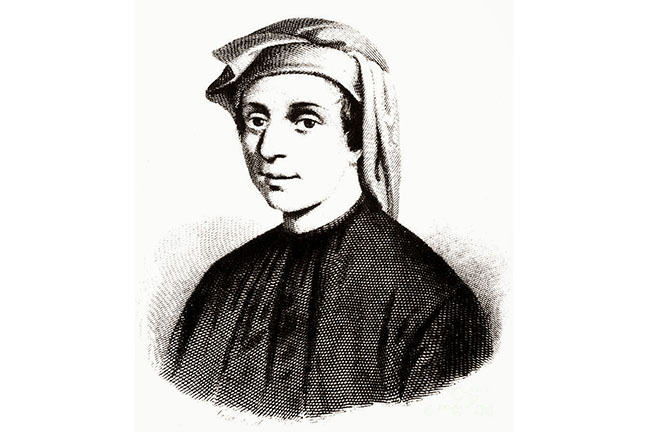## Want to keep learning?

This content is taken from the UNSW Sydney's online course, Maths for Humans: Linear, Quadratic & Inverse Relations. Join the course to learn more.
3.24

## UNSW SydneyFibonacci

# Composing Fibonacci's rabbits

How can we use matrix multiplication and Mobius transformations in a concrete example? Here we apply these ideas to investigate a classical problem that goes back to the medieval mathematician Fibonacci (1170- 1250), also known as Leonardo of Pisa. He was interested in how rabbits might reproduce.

In this step we learn about

• the Fibonacci sequence

• how to use matrix multiplication to predict future rabbit populations.

## Growth of rabbitsWild Rabbits at Edinburgh Zoo By Worm That Turned (Own work) CC BY-SA 3.0, via Wikimedia Commons

Fibonacci introduced an idealized model of how rabbits reproduce, and then used it to generate the sequence

$\Large 1, 1, 2, 3, 5, 8, 13, 21, 34, 55, 89, 144, 233, 377,....$

The pattern is that the first two numbers in the sequence are both $$\normalsize 1$$, and then any subsequent term is the sum of the two previous. This sequence has many remarkable properties, and also an intimate connection with the Golden ratio.

Fibonacci only computed the sequence up to the 14th term 377. But suppose we wanted to compute the 16th term in the sequence without knowing beforehand Fibonacci’s calculation. Or the 100th term? Could we find these in a more elegant fashion than working out all the terms in the sequence, one by one?

Curiously, we can answer this question by considering the Mobius transformation

$\Large f(x)=y=\frac{1}{x+1}$

and its associated matrix

$\Large F= \begin{pmatrix} 0 & 1\\ 1 & 1 \end{pmatrix}.$

Please get a feeling for why these are involved by working out some compositions.

Q1 (E): What do you get when you compose $$\normalsize f$$ with itself, in other words $$\normalsize f \circ f$$?

Q2 (M): How about $$\normalsize f \circ (f \circ f)$$?

Q3 (M): How about $$\normalsize (f \circ f) \circ (f \circ f))$$?

## Composing Mobius transformations or multiplying matrices

Please make sure you have done the three previous Questions, and/or looked at the answers. We start to suspect that the numerator and denominators of these various compositions just keep on involving higher and higher terms in the Fibonacci sequence. In fact any composition will involve exactly three consecutive terms.

Q4 (C): Can you give an argument for why this happens?

But we know that composing Mobius transformations is the same as multiplying matrices! So we can find the 16th power of $$\normalsize F$$ as follows. Time to practice our matrix multiplication! Observe that

$\Large F^2= \begin{pmatrix} 0 & 1\\ 1 & 1 \end{pmatrix}\begin{pmatrix} 0 & 1\\ 1 & 1 \end{pmatrix}= \begin{pmatrix} 1 & 1\\ 1 & 2 \end{pmatrix}$ $\Large F^4=(F^2)(F^2) = \begin{pmatrix} 1 & 1\\ 1 & 2 \end{pmatrix}\begin{pmatrix} 1 & 1\\ 1 & 2 \end{pmatrix}= \begin{pmatrix} 2 & 3\\ 3 & 5 \end{pmatrix}$ $\Large F^8=(F^4)(F^4) = \begin{pmatrix} 2 & 3\\ 3 & 5 \end{pmatrix}\begin{pmatrix} 2 & 3\\ 3 & 5 \end{pmatrix}= \begin{pmatrix} 13 & 21\\ 21 & 34 \end{pmatrix}$ $\Large F^{16}=(F^8)(F^8) = \begin{pmatrix} 13 & 21\\ 21 & 34 \end{pmatrix}\begin{pmatrix} 13 & 21\\ 21 & 34 \end{pmatrix}= \begin{pmatrix} 610 & 987\\ 987 & 1597 \end{pmatrix}.$

We see only Fibonacci numbers appearing in these matrix products. So for example the 4th Fibonacci number 3 is the top right hand corner of $$\normalsize F^{4}$$. Similarly the 16th Fibonacci number 987 appears in the top right corner of $$\normalsize F^{16}$$.

Q5 (M): Use this method to find the 32nd Fibonacci number.

Q6 (C): Use this method, and a bit of lateral thinking, to find the 100th Fibonacci number!

A1. A bit of algebra shows that

$\Large f \circ f = \frac{x+1}{x+2}.$

A2. A bit more algebra shows that

$\Large f \circ (f\circ f)=\frac{x+2}{2x+3}.$

A3. Yet more algebra shows that

$\Large (f \circ f) \circ (f \circ f)=\frac{2x+3}{3x+5}.$

A4. See discussion.

A5. The 32nd Fibonacci number is 2178309.

A6. The 100th Fibonacci number is… heh, why not ask Wolfram Alpha??